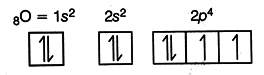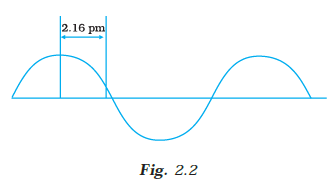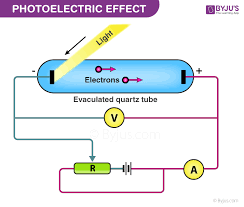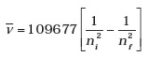Checkout JEE MAINS 2022 Question Paper Analysis : Checkout JEE MAINS 2022 Question Paper Analysis :

# Important Questions for Class 11 Chemistry Chapter 2 Structure of Atom.

Class 11 chemistry important questions with answers are provided here for Chapter 2 Structure of Atom. These important questions are based on the CBSE board curriculum and correspond to the most recent Class 11 chemistry syllabus. By practising these Class 11 important questions, students will be able to quickly review all of the ideas covered in the chapter and prepare for the Class 11 Annual examinations as well as other entrance exams such as NEET and JEE.

Download Class 11 Chemistry Chapter 2 Structure of Atom Important Questions with Answers PDF by clicking on the button below.

## Class 11 Structure of Atom Important Questions with Answers

Q1. Arrange s, p and d sub-shells of a shell in the increasing order of effective nuclear charge (Zeff) experienced by the electron present in them.

The increasing order of effective nuclear charge (Zeff) experienced by the electron present in them is s > p > d because s is close to the nucleus, and it will shield the nuclear charge more effectively.

Q2. Show the distribution of electrons in an oxygen atom (atomic number 8) using an orbital diagram.

The distribution of electrons in an oxygen atom using an orbital diagram is-From the above diagram, it can be concluded that oxygen has two unpaired electrons.

Q3. Nickel atom can lose two electrons to form Ni²+ ion. The atomic number of nickel is 28. From which orbital will nickel lose two electrons?

Ni atom’s electronic configuration is [Ar]18 3d8 4s2. It will lose two electrons from the 4s orbital to form Ni 2+ with the electronic configuration [Ar]18 3d8 4s0.

Q4. Which of the following orbitals are degenerate?

$$\begin{array}{l}3d_{xy}, 4d_{xy}, 3d_{z^{2}}, 3d_{yz}, 4d_{yz}, 4d_{z^{2}}\end{array}$$

Degenerate orbitals are those that have the same shells and subshells. Hence,

$$\begin{array}{l}3d_{xy}, 4d_{xy}, 3d_{z^{2}}, 3d_{yz}, 4d_{yz}, 4d_{z^{2}}\end{array}$$
are all degenerate orbitals.

Q5. Calculate the total number of angular nodes and radial nodes present in the 3p orbital.

For the 3p – orbital, the principal quantum number is n = 3 and the azimuthal number is l = 1.

Number of angular nodes will be l = 1

The number of radial nodes is equal to n – l – 1 = 3 – 1 – 1 = 1.

Q6. The arrangement of orbitals on the basis of energy is based upon their (n+l) value. Lower the value of (n+1), the lower is the energy. For orbitals having the same values of (n+l), the orbital with a lower value of n will have lower energy.

I. Based upon the above information, arrange the following orbitals in the increasing order of energy.

(a) 1s, 2s, 3s, 3p

(b) 4s, 3s, 3p, 4d

(c) 5p, 4d, 5d, 4f, 6s

(d) 5f, 6d, 7s, 7p

II. Based upon the above information, solve the questions given below :

(a) Which of the following orbitals has the lowest energy?

4d, 4f, 5s, 5p

(b) Which of the following orbitals has the highest energy?

5p, 5d, 5f, 6s, 6p

I.

1. 1s < 2s < 3s < 3p
2. 3s < 3p < 4s < 4d
3. 4d < 5p < 6s < 4f < 5d
4. 7s < 5f < 6d < 7p

II.

1. 5s has the lowest energy.
2. 5f has the highest energy.

Q7. Which of the following will not show deflection from the path on passing through an electric field?

Proton, cathode rays, electron, neutron.

Neutrons will not deviate from their path when passing through an electric field because they are neutral in nature and thus are unaffected by any electrical field.

Q8. An atom having atomic mass number 13 has 7 neutrons. What is the atomic number of the atom?

The atomic number can be calculated by

Atomic Number = Mass Number – Number of neutrons
Atomic Number = 13 – 7 = 6.

Hence, the atomic number will be 6.

Q9. Wavelengths of different radiations are given below:

λ(A) = 300 nm

λ(B) = 300 μm

λ (C) = 3 nm

λ = 0 (D) 30 Å

Arrange these radiations in the increasing order of their energies.

Since, E ∝ 1/ λ

Therefore, λ(B) < λ(A) < λ(C) = λ(D)

300μm < 300 nm < 3 nm = 30Å

Q10. The electronic configuration of the valence shell of Cu is 3d10 4s1 and not 3d9 4s2. How is this configuration explained?

This is explained by the orbital symmetry principle, which states that orbitals that are completely or partially filled in a given subshell have extra stability.

As a result, the 3d10 4s1 copper configuration is more symmetrical and stable than the 3d9 4s2 configuration.

Q11. The Balmer series in the hydrogen spectrum corresponds to the transition from n₁ = 2 to n₂ = 3,4,… This series lies in the visible region. Calculate the wave number of the line associated with the transition in the Balmer series when the electron moves to n = 4 orbit. (RH = 109677 cm-¹)

$$\begin{array}{l}=109677\left [ \frac{1}{\left ( n_{1} \right )^{2}}-\frac{1}{\left ( n_{2} \right )^{2}} \right ] cm^{-1}\end{array}$$

For n1 = 1, and n2 = 4

$$\begin{array}{l}=109677\left [ \frac{1}{\left ( 2 \right )^{2}}-\frac{1}{\left ( 4 \right )^{2}} \right ] cm^{-1}\end{array}$$

⊽ = 20564.44 cm–1.

Q12. According to de Broglie, the matter should exhibit dual behaviour, that is, both particle and wave like properties. However, a cricket ball of mass 100 g does not move like a wave when it is thrown by a bowler at a speed of 100 km/h. Calculate the wavelength of the ball and explain why it does not show wave nature.

According to de Broglie,

The wavelength =

$$\begin{array}{l}\lambda =\frac{h}{mv}\end{array}$$

m = 100 g = 0.1 kg.

$$\begin{array}{l}v=100 km/hr=\frac{100\times 1000}{60\times 60}=\frac{1000}{36} ms^{-1}\end{array}$$

h =6.626×10-34 Js

Therefore, in this case-

$$\begin{array}{l}\lambda =\frac{6.626\times 10^{-34}Js}{0.1kg\times \frac{1000}{36}ms^{-1}}\end{array}$$

λ = 238.5 × 10-36 m

Since the wavelength is too small to detect, it does not exhibit wave nature.

Q13. What is the experimental evidence in support of the idea that electronic energies in an atom are quantized?

The bright-line spectrum demonstrates that an atom’s energy levels are quantized. Classical mechanics cannot explain the behaviour of electrons, atoms, and molecules, among other things. This is due to the fact that classical mechanics ignores the concept of matter’s dual nature. Quantum mechanics takes these theories into account.

Q14. Out of electrons and protons which one will have a higher velocity to produce matter waves of the same wavelength? Explain it.

$$\begin{array}{l}\lambda =\frac{h}{mv}\end{array}$$

m1v1=m2v2 for two different particles with the same wavelength, i.e electron and proton (h is constant ). The particle’s velocity increases as its mass decreases. As a result, for the same wavelength, the electron will have a higher velocity because its mass is less than that of the proton.

Q15. A hypothetical electromagnetic wave is shown in Fig. Find out the wavelength of the radiation.The distance between two successive peaks or troughs of a wave is defined as its wavelength. So λ = 4 × 2.16 pm = 8.64 pm.

Q16. Chlorophyll present in green leaves of plants absorbs light at 4.620 x 1014 Hz. Calculate the wavelength of radiation in nanometer. Which part of the electromagnetic spectrum does it belong to?

Given: 𝜈 = 4.620 x 1014 Hz or 4.620 x 1014 s-1.

$$\begin{array}{l}\lambda =\frac{c}{\nu}\end{array}$$
$$\begin{array}{l}\lambda =\frac{c}{\nu }=\frac{3\times 10^{8}ms^{-1}}{4.620\times 10^{14}s^{-1}}\end{array}$$

λ = 0.6494 x 10-6 m = 649.4 nm.

Thus, it belongs to the visible region.

Q17. What is the difference between the terms orbit and orbital?

Orbit Orbitals
An orbit is the simple planar representation of an electron. An orbital refers to the dimensional motion of an electron around the nucleus in a three-dimensional motion.
It can be simply defined as the path that gets established in a circular motion by revolving the electron around the nucleus An orbital can simply be defined as the space or the region where the electron is likely to be found the most.
The shape of molecules cannot be explained by an orbit as they are non-directional by nature. The shapes of the molecules can be found as they are directional by nature.
An orbit that is well-defined goes against the Heisenberg principle. An ideal orbital agrees with the theory of Heisenberg’s Principles.

Q18. Table-tennis ball has a mass of 10 g and a speed of 90 m/s. If speed can be measured with an accuracy of 4% what will be the uncertainty in speed and position?

Given: speed = 90 m/s, mass = 10 g = 10 × 10-3 kg.

Uncertainty in speed (Δv) = 4% of 90 m/sec =

$$\begin{array}{l}\frac{4\times 90}{100}= 36m/sec\end{array}$$
$$\begin{array}{l}\Delta x.\Delta v=\frac{h}{4\Pi m}\end{array}$$
From Heisenberg Uncertainty Principle

$$\begin{array}{l}\Delta z=\frac{h}{4\Pi m\times \Delta v}\end{array}$$
$$\begin{array}{l}\Delta z=\frac{6.626\times 10^{-34}Js}{4\times 3.14\times 10\times 10^{-3}kg\times 36ms^{-1}}\end{array}$$

Δx = 1.46 × 10-33 m.

Q19. The effect of the uncertainty principle is significant only for the motion of microscopic particles and is negligible for macroscopic particles. Justify the statement with the help of a suitable example.

The uncertainty principle has no practical application in our daily lives, i.e. in the macroscopic world. It is because a photon’s energy is insufficient to change the position and velocity of macroscopic objects. Only in the case of smaller moving particles such as electrons does the uncertainty principle come into play.

$$\begin{array}{l}\Delta x.\Delta v=\frac{h}{4\Pi m}\end{array}$$

If the Heisenberg uncertainty principle is to be applied on an object with a mass of about a milligram, then,

$$\begin{array}{l}\Delta x.\Delta v=\frac{6.626\times 10^{-34}Js}{4\times 3.14\times10^{-6}kg}\end{array}$$
$$\begin{array}{l}\Delta x.\Delta v=0.52\times 10^{-28}Js\end{array}$$

The value of Δx.Δv obtained is very small and insignificant. As a result, for milligram-sized or heavier objects, the associated uncertainties are hardly significant.

Q20. A hydrogen atom has only one electron, so mutual repulsion between electrons is absent. However, in multielectron atoms mutual repulsion between the electrons is significant. How does this affect the energy of an electron in the orbitals of the same principal quantum number in multielectron atoms?

The principal quantum number determines the energy of an electron in a hydrogen atom. As a result, the energy of the orbitals increases in the following way:

1s < 2s = 2p < 3s = 3p = 3d < 4s = 4p = 4f < (2.23)

Unlike the hydrogen atom, the energy of an electron in a multielectron atom is determined not only by its principal quantum number (shell) but also by its azimuthal quantum number (subshell). That is, for a given principal quantum number, the energies of s,p,d,f…. are all different.

### Matching Type Questions

In some of the following questions, one option in the left column may be correlated to more than one option in the right column.

Q1. Match the following species with their corresponding ground state electronic configuration.

Atom / Ion Electronic configuration
(i) Cu (a) 1s2 2s2 2p6 3s2 3p6 3d10
(ii) Cu²+ (b) 1s2 2s2 2p6 3s2 3p6 3d10 4s2
(iii) Zn2+ (c) 1s2 2s2 2p6 3s2 3p6 3d10 4s1
(iv) Cr3+ (d) 1s2 2s2 2p6 3s2 3p6 3d9
(e) 1s2 2s2 2p6 3s2 3p6 3d3

Atom / Ion Electronic configuration
(i) Cu (c) 1s2 2s2 2p6 3s2 3p6 3d10 4s1
(ii) Cu²+ (d) 1s2 2s2 2p6 3s2 3p6 3d9
(iii) Zn2+ (a) 1s2 2s2 2p6 3s2 3p6 3d10
(iv) Cr3+ (e) 1s2 2s2 2p6 3s2 3p6 3d3

Q2. Match the quantum numbers with the information provided by these.

Quantum number Information provided
(i) Principal quantum number (a) orientation of the orbital
(ii) Azimuthal quantum number (b) energy and size of orbital
(iii) Magnetic quantum number (c) spin of electron
(iv) Spin quantum number (d) shape of the orbital

Quantum number Information provided
(i) Principal quantum number (b) energy and size of orbital
(ii) Azimuthal quantum number (d) shape of the orbital
(iii) Magnetic quantum number (a) orientation of the orbital
(iv) Spin quantum number (c) spin of electron

Q3. Match the following rules with their statements:

Rules Statements
(i) Hund’s Rule (a) No two electrons in an atom can have the same set of four quantum numbers.
(ii) Aufbau Principle (b) Half-filled and completely filled orbitals have extra stability.
(iii) Pauli Exclusion Principle (c) Pairing of electrons in the orbitals belonging to the same subshell does not take place until each orbital is singly occupied.
(iv) Heisenberg’s Uncertainty Principle (d) It is impossible to determine the exact position and exact momentum of a subatomic particle simultaneously.
(e) In the ground state of atoms, orbitals are filled in the order of their increasing energies.

Rules Statements
(i) Hund’s Rule (c) Pairing of electrons in the orbitals belonging to the same subshell does not take place until each orbital is singly occupied.
(ii) Aufbau Principle (e) In the ground state of atoms, orbitals are filled in the order of their increasing energies.
(iii) Pauli Exclusion Principle (a) No two electrons in an atom can have the same set of four quantum numbers.
(iv) Heisenberg’s Uncertainty Principle (d) It is impossible to determine the exact position and exact momentum of a subatomic particle simultaneously.

Q4. Match the following

(i) X-rays (a) v = 100-104 Hz
(ii) UV (b) v = 1010 Hz
(iii) Long radio waves (c) v = 1016 Hz
(iv) Microwave (d) v = 1018 Hz

(i) X-rays (d) v = 1018 Hz
(ii) UV (c) v = 1016 Hz
(iii) Long radio waves (a) v = 100-104 Hz
(iv) Microwave (b) v = 1010 Hz

Q5. Match the following

(i) Photon (a) Value is 4 for N shell
(ii) Electron (b) Probability density
(iii) Ψ2 (c) Always positive value
(iv) Principal quantum number n (d) Exhibits both momentum and wavelength

(i) Photon (d) Exhibits both momentum and wavelength
(ii) Electron (d) Exhibits both momentum and wavelength
(iii) Ψ2 (b) Probability density (c) Always positive value
(iv) Principal quantum number n (a) Value is 4 for N shell (c) Always positive value

Q6. Match species given in Column I with the electronic configuration given in Column II.

Column I Column II
(i) Cr (a) [Ar]3d8 4s0
(ii) Fe2+ (b) [Ar]3d10 4s1
(iii) Ni2+ (c) [Ar]3d6 4s0
(iv) Cu (d) [Ar]3d5 4s1
(e) [Ar]3d6 4s2

Column I Column II
(i) Cr (d) [Ar]3d5 4s1
(ii) Fe2+ (c) [Ar]3d6 4s0
(iii) Ni2+ (a) [Ar]3d8 4s0
(iv) Cu (b) [Ar]3d10 4s1

### Assertion and Reason Type Questions

In the following questions a statement of Assertion (A) followed by a statement of Reason (R) is given. Choose the correct option out of the choices given below each question.

Q1. Assertion (A): All isotopes of a given element show the same type of chemical behaviour. Reason (R): The chemical properties of an atom are controlled by the number of electrons in the atom.

1. Both A and R are true and R is the correct explanation of A.
2. Both A and R are true but R is not the correct explanation of A.
3. A is true but R is false.
4. Both A and R are false.

Correct Answer is (1.) Both A and R are true and R is the correct explanation of A.

All isotopes have the same atomic number, which means they have the same number of electrons. So, they bear similar chemical properties.

Q2. Assertion (A): Black body is an ideal body that emits and absorbs radiation of all frequencies.

Reason (R): The frequency of radiation emitted by a body goes from a lower frequency to a higher frequency with an increase in temperature.

1. Both A and R are true and R is the correct explanation of A.
2. Both A and R are true but R is not the explanation of A
3. A is true and R is false;
4. Both A and R are false.

Correct Answer is (2.) Both A and R are true but R is not the correct explanation of A.

A black body is an ideal body that emits and absorbs radiation of all frequencies, and the radiation emitted by such a body is known as black body radiation. The exact frequency distribution of a black body’s emitted radiation is determined solely by its temperature. At a given temperature, the intensity of radiation emitted increases as the wavelength decreases, reaches a maximum at a given wavelength, and then begins to decrease as the wavelength decreases further.

Q3. Assertion (A): It is impossible to determine the exact position and exact momentum of an electron simultaneously.

Reason (R): The path of an electron in an atom is clearly defined.

1. Both A and R are true and R is the correct explanation of A.
2. Both A and R are true and R is not the correct explanation of A.
3. A is true and R is false.
4. Both A and R are false.

Correct Answer is (3.) A is true and R is false.

The exact position and exact momentum of an electron cannot be determined simultaneously, according to Heisenberg’s uncertainty principle. As a result, the electron’s path in an atom is not well defined.

Q1. What is the photoelectric effect? State the result of a photoelectric effect experiment that could not be explained on the basis of laws of classical physics. Explain this effect on the basis of quantum theory of electromagnetic radiation.

The photoelectric effect occurs when certain metals are exposed to a light beam, causing electrons to be ejected from the metal. This is known as the photoelectric effect. The ejected electrons are known as photoelectrons.This experiment yielded the following results:

1. Electrons are ejected from the metal surface only when a light beam strikes it.
2. The number of electrons that make up the intensity of radiation.
3. There is a minimum frequency, v0, for each metal below which the photoelectric effect is not observed.
4. K.E. of electrons α frequency of light.

Explanation of the photoelectric effect based on quantum theory: When a sufficiently energetic photon collides with an electron in a metal atom, the photon transfers its energy to the electron, and the electron is ejected without delay. The higher the energy of the photon, the higher the kinetic energy of the ejected electron.

Q2. Threshold frequency, Vo is the minimum frequency that a photon must possess to eject an electron from a metal. It is different for different metals. When a photon of frequency 1.0 x 1015 s-1 was allowed to hit a metal surface, an electron having 1.988 x 10–19 J of kinetic energy was emitted. Calculate the threshold frequency of this metal. Show that an electron will not be emitted if a photon with a wavelength equal to 600 nm hits the metal surface.

hv = h0 + K.E

hv0 = hv – K.E

$$\begin{array}{l}v_{0}=v-\frac{K.E}{h}\end{array}$$

v = 1.0 × 1015 s–1

K.E. = 1.988 × 10-19 J, h = 6.626 × 10–34 Js

$$\begin{array}{l}v_{0}=(1.0\times 10^{15}s^{-1})-\frac{1.988\times 10^{-19}J}{6.626\times 10^{-34}Js}\end{array}$$
$$\begin{array}{l}v_{0}=1.0\times 10^{15}s^{-1}-0.30\times 10^{-15}s^{-1}\end{array}$$

v0 = 7 × 1014 s–1

λ = 600 nm = 600 × 10-9 m = 6.0 × 10-7 m

$$\begin{array}{l}v=\frac{c}{\lambda }=\frac{3.0\times 10^{8}m s^{-1}}{6\times 10^{-7}m}=5\times 10^{14}s^{-1}\end{array}$$

Thus, v < v0 and therefore, no electron will be emitted.

Q3. When an electric discharge is passed through hydrogen gas, the hydrogen molecules dissociate to produce excited hydrogen atoms. These excited atoms emit electromagnetic radiation of discrete frequencies which can be given by the general formulaWhat points of Bohr’s model of an atom can be used to arrive at this formula? Based on these points, derive the above formula giving a description of each step and each term.

The following are the two key points of Bohr’s model that can be used to derive the given formula:

(i) Electrons orbit the nucleus in a circular path with a fixed radius and energy. Orbits, stationary states, and allowed energy states are all names for these paths.

(ii) When an electron moves from a higher stationary state to a lower stationary state or from a lower stationary state to a higher stationary state, energy is emitted or absorbed. The equation gives the energy difference between the two orbits.

ΔE = E2 – E1

When an electron jumps from outer orbit n2 to inner orbit n1, the difference of energy (ΔE) is emitted.

$$\begin{array}{l}\Delta E = E_{2}-E_{1}=\frac{-2\Pi ^{2}me^{4}}{n_{2}^{2}h^{2}}-(\frac{-2\Pi ^{2}me^{4}}{n_{1}^{2}h^{2}})\end{array}$$
$$\begin{array}{l}\Delta E = E_{2}-E_{1}=\frac{-2\Pi ^{2}me^{4}}{h^{2}}\left ( \frac{1}{n_{1}^{2}}-\frac{1}{n_{2}^{2}} \right )\end{array}$$

⊽ = ΔE/hc

$$\begin{array}{l}=\frac{-2\Pi ^{2}me^{4}}{ch^{2}}\left ( \frac{1}{n_{1}^{2}}-\frac{1}{n_{2}^{2}} \right )\end{array}$$

By putting the values of π, m, c, h and e we get-

⊽ =

$$\begin{array}{l}109677\left( \frac{1}{n_{1}^{2}}-\frac{1}{n_{2}^{2}} \right )cm^{-1}\end{array}$$

Q4. Calculate the energy and frequency of the radiation emitted when an electron jumps from n = 3 to n = 2 in a hydrogen atom.

n2 = 3, n1 = 2

⊽ =

$$\begin{array}{l}109677\left( \frac{1}{n_{1}^{2}}-\frac{1}{n_{2}^{2}} \right )cm^{-1}\end{array}$$
$$\begin{array}{l}=109677\left( \frac{1}{2^{2}}-\frac{1}{3^{2}} \right )cm^{-1}\end{array}$$
$$\begin{array}{l}=109677\times \frac{5}{36}=15232.9 cm^{-1}\end{array}$$

λ = 1/⊽

$$\begin{array}{l}\lambda =\frac{1}{15232.9}=6.564\times 10^{-5}cm=6.564\times 10^{-7}m\end{array}$$
$$\begin{array}{l}E=\frac{hc}{\lambda }=\frac{6.6\times 10^{-34}Js\times 3\times 10^{8}ms^{-1}}{6.564\times 10^{-7}m}\end{array}$$
$$\begin{array}{l}E=3.028\times 10^{-19}J\end{array}$$

Frequency 𝜈 = c/λ

$$\begin{array}{l}\nu =\frac{3\times 10^{8}ms^{-1}}{6.56\times 10^{-7}}=0.457\times 10^{15}s\end{array}$$

𝜈 = 4.57 × 1014 s-1.

Q5. Why was a change in the Bohr Model of atom required? Due to which important development (s), the concept of movement of an electron in an orbit was replaced by, the concept of probability of finding an electron in an orbital? What is the name given to the changed model of the atom?

A modification to the Bohr model was required due to certain defects. The Bohr model is applied to atoms with a single electron. It cannot account for multi-electron atoms. It also claims that electrons move in circular orbits around the nucleus, which is incorrect.

The dual behaviour of matter and the Heisenberg uncertainty principle were two significant developments that contributed significantly to the formulation of such a model.

In 1972, Werner Heisenberg, a German physicist, stated the uncertainty principle, which is the result of the dual behaviour of matter and radiation. The existence of definite paths or trajectories of electrons and other similar particles is governed by the Heisenberg Uncertainty Principle, which is one of its most important implications.

Quantum mechanics is a theoretical science that studies the motions of microscopic objects that have both observable wave and particle properties. The new atom model is known as the Quantum Mechanical Model of Atom.

Test your Knowledge on Important questions class 11 chemistry chapter 2 structure atom!